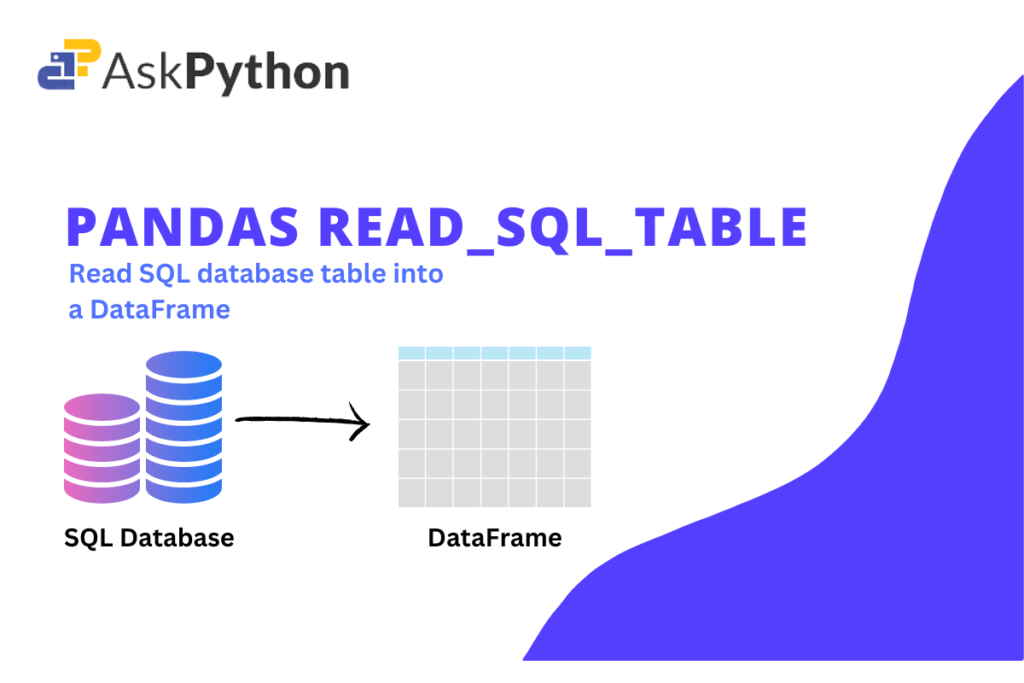You might always wonder, where does such huge amount of data generated in different fields, different sectors, different companies stores?Well, the answer is all the data is stored in databases using SQL(Structured Query Languages) in form of different tables. You can refer to know more about databases or the language SQL. So now for further data analysis we need to covert these SQL database tables into Pandas DataFrames and that’s where the function ‘read_sql_table’ plays a vital role.

## Syntax of pandas read_sql_table() function

```pandas.read_sql_table(table_name, con, schema=None, index_col=None, coerce_float=True, parse_dates=None, columns=None, chunksize=None)
```

Some of the most used parameters are mentioned below.

For more details about different parameters refer to the official documentation.

## Creating database and table using SQAlchemy

Before we go into learning how to use pandas read_sql_table() and other functions, let’s create a database and table by using `sqlite3`.

The example below shows how to use the sqlalchemy library to create a database and table in Python. Install a sqlalchemy library if you don’t already have one by using the pip command. You must first import it before you can use it.

```from sqlalchemy import MetaData
```

As a result, an object of the MetaData class from SQLAlchemy is created. Metadata is a collection of Table objects and the schema constructs that go with them. It contains a set of Table objects, as well as an optional binding to an Engine or Connection.

### Creating table into database

Using Table module from sqlalchemy, you can easily create a table specifying the column name with their data type for e.g ‘name’ with String datatype. Also before creating a table, you have to create a database by using create_engine function. Once the engine variable is created, create_all function from meta is used to store the given Table class variables.

```from sqlalchemy import Table, Column, Integer, String, MetaData, create_engine
#creates a database
engine = create_engine('sqlite:///school.db', echo = True)
#creates a table
students = Table(
'students', meta,
Column('id', Integer, primary_key = True),
Column('name', String),
Column('rank', Integer),
)

meta.create_all(engine)
```

Also read: How to insert multiple records using SQLite3

### Inserting rows into created table

After connecting to database, you can insert rows into the table now by using the connect object’s execute() function. Here, student.insert() is interpreted by the connect object as a SQL query given below:

```INSERT INTO STUDENTS VALUES(1,'Arun',1)
```

Here is small code snippet of inserting 5 rows into the student table:

```#connect to the database
conn = engine.connect()
#executing sql query
conn.execute(students.insert(), [
{'id':1,'name':'Arun','rank':1},
{'id':2, 'name':'Komal','rank':2},
{'id':3,'name':'Abdul','rank':3},
{'id':4,'name':'Priya','rank':4},
{'id':5,'name':'Ranjan','rank':2}
])
```

There are many ways to create a SQL database and a table using python like Sqlite3. For more details visit:

## Converting the SQL table into dataframe using Pandas read_sql_table()

As previously stated, the pandas read_sql_table() function can take either a SQL query or a table name as a parameter. After connecting to the database using connect function, we can call the read_sql_table function with first parameter as the table name and next the connect variable.

```# SQLAlchemy connectable
cnx = engine.connect()

# table named 'students' will be returned as a dataframe.
print(df)
```
```In this tutorial, you learned about the Pandas read_sql_table() function which enables the user to read a SQL query into a Pandas DataFrame. You also saw examples that demonstrated how to create a database, add a table to it, display the records from the table, and also how to connect databases using SQLalchemy.